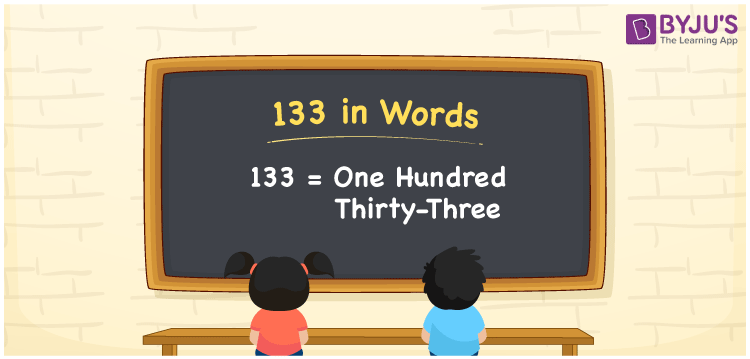# 133 in Words

133 in words is written as One hundred thirty-three. In both the International System of Numerals and the Indian System of Numerals, 133 is written as One hundred thirty-three. The number 133 is a Cardinal Number as it represents some quantity. For example, “there are 133 people in the room”.

 133 in Words One hundred thirty-three One hundred thirty-three in Number 133

## 133 in English Words

We write 133 in English Words using the letters of the English alphabet. Therefore, we read 133 in English as “One hundred thirty-three.”## How to Write 133 in Words?

To write 133 in words, we shall use the place value chart. In the place value chart, write 1 in the hundreds, 3 in the tens, and 3 in the ones, respectively. Now let us make a place value chart to write the number 133 in words.

 Hundreds Tens Ones 1 3 3

Thus, we can write the expanded form as

1 × Hundred + 3 × Ten + 3 × One

= 1 × 300 + 3 × 30 + 3 × 1

= 100 + 30 + 3

= 133

= One hundred thirty-three.

133 is a natural number, the successor of 132 and the predecessor of 134.

133 in words – One hundred thirty-three

• Is 133 an odd number? – Yes
• Is 133 an even number? – No
• Is 133 a perfect square number? – No
• Is 133 a perfect cube number? – No
• Is 133 a prime number? – No
• Is 133 a composite number? – Yes

## Frequently Asked Questions on 133 in Words

Q1

### How to write 133 in words?

133 in words is written as One hundred thirty-three.
Q2

### How to write 133 in the International and Indian System of Numerals?

In both, the system of numerals, 133 in words, is written as One hundred thirty-three.
Q3

### What are the factors of 133?

The factors of 133 are 1, 7, 19 and 133.